International
Tables for
Crystallography
Volume B
Reciprocal space
Edited by U. Shmueli

International Tables for Crystallography (2010). Vol. B, ch. 1.4, pp. 115-116   | 1 | 2 |

## Section 1.4.2.2. Relationship between structure factors at symmetry-related points of the reciprocal lattice

U. Shmuelia

#### 1.4.2.2. Relationship between structure factors at symmetry-related points of the reciprocal lattice

| top | pdf |

Of main interest in the context of the present chapter are symmetry relationships that concern the values of a function defined at the points of the reciprocal lattice. Such functions, of crystallographic interest, are Fourier-transform representations of direct-space functions that have the periodicity of the crystal, the structure factor as a Fourier transform of the electron-density function being a representative example (see e.g. Lipson & Taylor, 1958). The value of such a function, attached to a reciprocal-lattice point, is called the weight of this point and the set of all such weighted points is often termed the weighted reciprocal lattice. This section deals with a fundamental relationship between functions (weights) associated with reciprocal-lattice points, which are related by point-group symmetry, the weights here considered being the structure factors of Bragg reflections (cf. Chapter 1.2).

The electron density, an example of a three-dimensional periodic function with the periodicity of the crystal, can be represented by the Fourier serieswhere h is a reciprocal-lattice vector, V is the volume of the (direct) unit cell,is the structure factor at the point h and r is a position vector of a point in direct space, at which the density is given. The summation in (1.4.2.1) extends over all the reciprocal lattice.

Letbe a space-group operation on the crystal, where P and t are its rotation and translation parts, respectively, and P must therefore be a point-group operator. We then have, by definition,and the Fourier representation of the electron density, at the equivalent position, is given by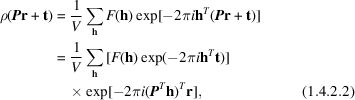noting that. Since P is a point-group operator, the vectorsin (1.4.2.2) must range over all the reciprocal lattice and a comparison of the functional forms of the equivalent expansions (1.4.2.1)and (1.4.2.2) shows that the coefficients of the exponentialsin (1.4.2.2)must be the structure factors at the pointsin the reciprocal lattice. Thuswherefrom it follows that the magnitudes of the structure factors at h andare the same:and their phases are related byThe relationship (1.4.2.3)between structure factors of symmetry-related reflections was first derived by Waser (1955),starting from a representation of the structure factor as a Fourier transform of the electron-density function.

It follows that an application of a point-group transformation to the (weighted) reciprocal lattice leaves the moduli of the structure factors unchanged. The distribution of diffracted intensities obeys, in fact, the same point-group symmetry as that of the crystal. If, however, anomalous dispersion is negligibly small, and the point group of the crystal is noncentrosymmetric, the apparent symmetry of the diffraction pattern will also contain a false centre of symmetry and, of course, all the additional elements generated by the inclusion of this centre. Under these circumstances, the diffraction pattern from a single crystal may belong to one of the eleven centrosymmetric point groups, known as Laue groups (IT I, 1952).

According to equation (1.4.2.5),the phases of the structure factors of symmetry-related reflections differ, in the general case, by a phase shift that depends on the translation part of the space-group operation involved. Only when the space group is symmorphic, i.e. it contains no translations other than those of the Bravais lattice, will the distribution of the phases obey the point-group symmetry of the crystal. These phase shifts are considered in detail in Section 1.4.4where their tabulation is presented and the alternative interpretation (Bienenstock & Ewald, 1962) of symmetry in reciprocal space, mentioned in Section 1.4.1, is given.

Equation (1.4.2.3)can be usefully applied to a classification of all the general systematic absences or – as defined in the space-group tables in the main editions of IT (1935, 1952, 1983, 1987, 1992, 1995, 2002) – general conditions for possible reflections. These systematic absences are associated with special positions in the reciprocal lattice – special with respect to the point-group operations P appearing in the relevant relationships. If, in a given relationship, we have, equation (1.4.2.3)reduces toOf course,may then be nonzero only ifequals unity, or the scalar productis an integer. This well known result leads to a ready determination of lattice absences, as well as those produced by screw-axis and glide-plane translations, and is routinely employed in crystallographic computing. An exhaustive classification of the general conditions for possible reflections is given in the space-group tables (IT, 1952, 1983). It should be noted that since the axes of rotation and planes of reflection in the reciprocal lattice are parallel to the corresponding elements in the direct lattice (Buerger, 1960), the component of t that depends on the location of the corresponding space-group symmetry element in direct space does not contribute to the scalar productin (1.4.2.6), and it is only the intrinsic part of the translation t (IT A, 1983) that usually matters.

It may, however, be of interest to note that some screw axes in direct space cannot give rise to any systematic absences. For example, the general Wyckoff position No. (10) in the space group(No. 205) (IT A, 1983) has the coordinates, and corresponds to the space-group operation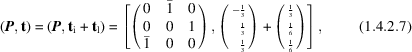where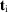and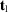are the intrinsic and location-dependent components of the translation part t, and are parallel and perpendicular, respectively, to the threefold axis of rotation represented by the matrix P in (1.4.2.7)(IT A, 1983; Shmueli, 1984). This is clearly a threefold screw axis, parallel to. The reciprocal-lattice vectors which remain unchanged, when postmultiplied by P (or premultiplied by its transpose), have the form:; this is the special position for the present example. We see that (i)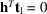, as expected, and (ii)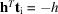. Since the scalar productis an integer, there are no values of index h for which the structure factormust be absent.

Other approaches to systematically absent reflections include a direct inspection of the structure-factor equation (Lipson & Cochran, 1966), which is of considerable didactical value, and the utilization of transformation properties of direct and reciprocal base vectors and lattice-point coordinates (Buerger, 1942).

Finally, the relationship between the phases of symmetry-related reflections, given by (1.4.2.5), is of fundamental as well as practical importance in the theories and techniques of crystal structure determination which operate in reciprocal space (Part 2of this volume).

### References

International Tables for Crystallography (1983). Vol. A, Space-Group Symmetry, edited by Th. Hahn. Dordrecht: Reidel.
International Tables for Crystallography (1987). Vol. A, Space-Group Symmetry, edited by Th. Hahn, 2nd ed. Dordrecht: Reidel.
International Tables for Crystallography (1992). Vol. A, Space-Group Symmetry, edited by Th. Hahn, 3rd ed. Dordrecht: Kluwer Academic Publishers.
International Tables for Crystallography (1995). Vol. A, Space-Group Symmetry, edited by Th. Hahn, 4th ed. Dordrecht: Kluwer Academic Publishers.
International Tables for Crystallography (2002). Vol. A, Space-Group Symmetry, edited by Th. Hahn, 5th ed. Dordrecht: Kluwer Academic Publishers.
International Tables for X-ray Crystallography (1952). Vol. I, Symmetry Groups, edited by N. F. M. Henry & K. Lonsdale. Birmingham: Kynoch Press.
Internationale Tabellen zur Bestimmung von Kristallstrukturen (1935). 1. Band. Edited by C. Hermann. Berlin: Borntraeger.
Bienenstock, A. & Ewald, P. P. (1962). Symmetry of Fourier space. Acta Cryst. 15, 1253–1261.
Buerger, M. J. (1942). X-ray Crystallography. New York: John Wiley.
Buerger, M. J. (1960). Crystal Structure Analysis. New York: John Wiley.
Lipson, H. & Cochran, W. (1966). The Crystalline State, Vol. II, The Determination of Crystal Structures. London: Bell.
Lipson, H. & Taylor, C. A. (1958). Fourier Transforms and X-ray Diffraction. London: Bell.
Shmueli, U. (1984). Space-group algorithms. I. The space group and its symmetry elements. Acta Cryst. A40, 559–567.
Waser, J. (1955). Symmetry relations between structure factors. Acta Cryst. 8, 595.